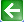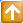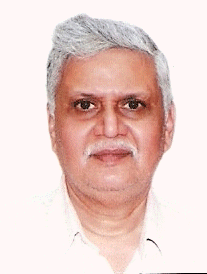Satish Lele lelepiping@gmail.com

Mass Balance
Definition: The accounting of all mass in a chemical / pharmaceutical process is referred to as mass (or material) balance. Similarly Energy accounting is referred to energy balance.
Uses: It is used in day to day operation of process for monitoring operating efficiency. It helps in making calculations for design and development of a process like quantities required, sizing equipment, number of items of equipment.
Example of Batch mixing process: 200 kg of a 40% w/w methanol / water solution is mixed with 100 kg of a 70% w/w methanol/water solution in a batch mixer unit. What is the final quantity and composition?
Total initial mass = total final mass = 300 kg. Initial methanol mass = final methanol mass. 80 + 70 = final methanol mass = 150 kg. Therefore final composition of batch is (150/300) x 100 = 50 % by wt.

Batch processes: Batch processes operate to a batch cycle and are non-steady state. Materials are added to a vessel in one operation and then process is carried out and batch cycle repeated. Integral balances are carried out on batch processes where balances are carried out on the initial and final states of the system.
Batch cycle: It depends on

• Sequence of operations/steps repeated according to a cycle
• Batch cycle time
• Batch size
Simple batch reaction cycle: In this there are 3 steps.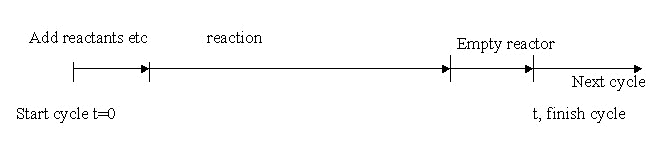Continuous processes: These processes are continuous in nature and operate in steady state and balances are carried out over a fixed period of time. Materials enter and leave process continuously.
Law of conservation of mass: When there is no net accumulation or depletion of mass in a system (steady state) then:
Total mass entering system = total mass leaving system or total mass at start = total final mass
General mass balance equation: Input + generation – output – consumption = accumulation.
Notes:
1. Generation and consumption terms refer only to generation of products and consumption of reactants as a result of chemical reaction. If there is no chemical reaction then these terms are zero.
2. Apply to a system.
3. Apply to total mass and component mass.
Definitions:
System – It is an arbitrary part or whole of a system.
Steady state/non-steady state.
Accumulation/depletion of mass in system.
Basis for calculation of mass balance (unit of time, batch etc).
Component or substance
Mixing of streams: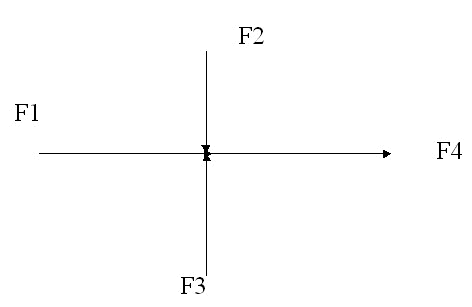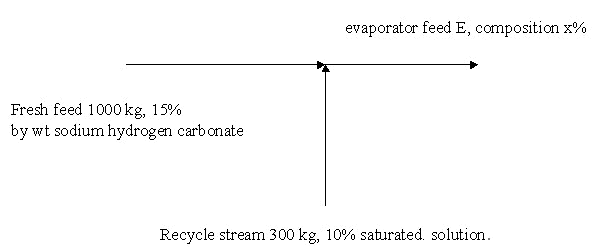Flow Sheets: It consists of Process flow diagram and PID.
• Streams:
• Operations/equipment sequence:
• Standard symbols:
Typical simple flow Sheet arrangement: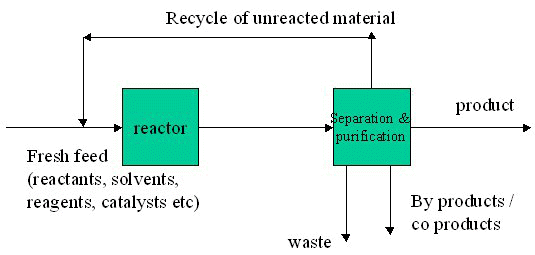Exercise: A 1000 kg batch of a pharmaceutical powder containing 5% by wt water is dried in a double cone drier. After drying 90% of the water has been removed. Calculate the final batch composition and the weight of water removed.
Exercise Batch Distillation: 1000 kg of a 20% by wt mixture of acetone in water is separated by multistage batch distillation. The top product (distillate) contains 95% by wt. acetone and the still contains 2% acetone. Calculate the amount of distillate.
Use of molar quantities: It is often useful to calculate a mass balance using molar quantities of materials and to express composition as mole fractions or mole %. Distillation is an example, where equilibrium data is often expressed in mole fractions.
Molar units:
• A mole is the molecular weight of a substance expressed in grams.
• To get the molecular weight of a substance you need its molecular formula and you can then add up the atomic weights of all the atoms in the molecule.
• To convert from moles of a substance to grams multiply by the molecular weight.
• To convert from grams to moles divide by the molecular weight.
• Mole fraction is moles divided by total moles.
• Mole % is mole fraction multiplied by 100.
Molar units: Benzene is C6H6. The molecular weight is (6x12) + (6x1) = 78. So 1 mole of benzene is 78 grams. 1 kilo mol is 78 kg.
Exercise of Batch Distillation: 1000 kilo mol of an equimolar mixture of benzene and toluene is distilled in a multistage batch distillation unit. 90% of the benzene is in the top product (distillate). The top product has a benzene mole fraction of 0.95. Calculate the quantities of top and bottom products and the composition of the bottom product.
 Temp oC Solubility gm KCl/100 gm water 80 51.1 70 48.3 60 45.5 50 42.6 40 40 30 37 20 34 10 31 0 27.6

Mass Balance of Crystallizer: A crystallizer contains 1000 kg of a saturated solution of potassium chloride at 80oC. It is required to crystallize 100 kg KCl from this solution. To what temperature must the solution be cooled?
At 80oC saturated solution contains (51.1/151.1) x 100% of KCl. It is 33.8% by weight. So in 1000 kg there is 338 kg KCl & 662 kg water. Crystallizing 100 kg out of solution leaves a saturated solution containing 238 kg KCl and 662 kg water, which is 238/6.62 gm KCl/100 gm water which is 36 gm KCl/100 gm. So temperature required is approx 27oC from table.

Mass Balance: filtration/centrifuge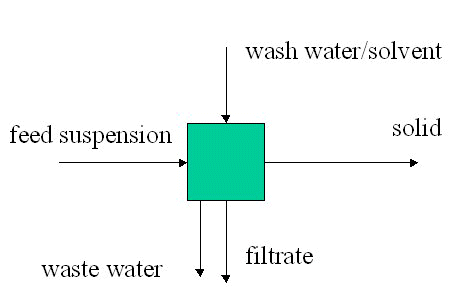Mass Balance: Filtration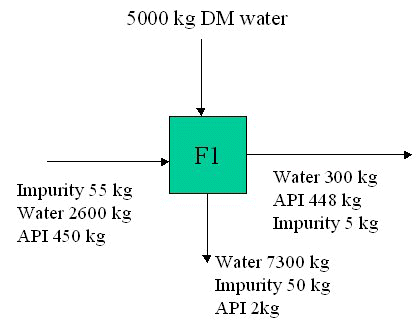Mass Balance Dryer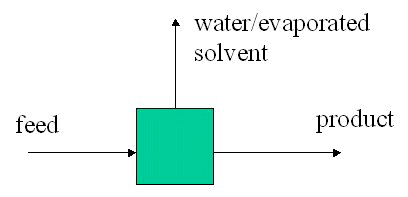Mass Balance: extraction/phase split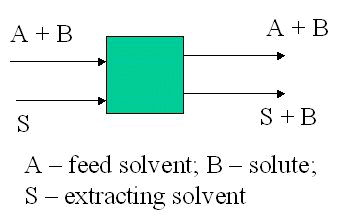Example (single stage extraction; immiscible solvents)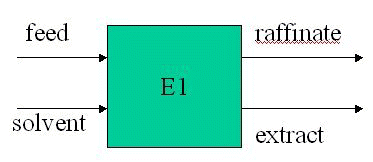F = 195 kg; xf = 0.11 kg API/kg water.
S = 596 kg chloroform.
y = 1.72x, where y is kg API/kg chloroform in extract and x is kg API/kg water in raffinate.

Total balance 195 + 596 = E + R
API balance 19.5 = 175.5x1 + 596y1
19.5 = 175.5x1 + 596.1.72x1
x1 = 0.0162 and y1 = 0.029
R is 175.5 kg water + 2.84 kg API
and E is 596 kg chloroform + 17.28 kg API

Note: chloroform and water are essentially immiscible
Mass balance – absorption unit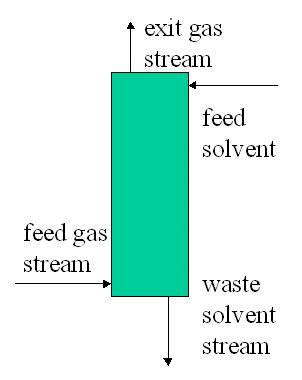Mass Balances of Multiple Units
• Overall balance
• Unit balances
• Component balances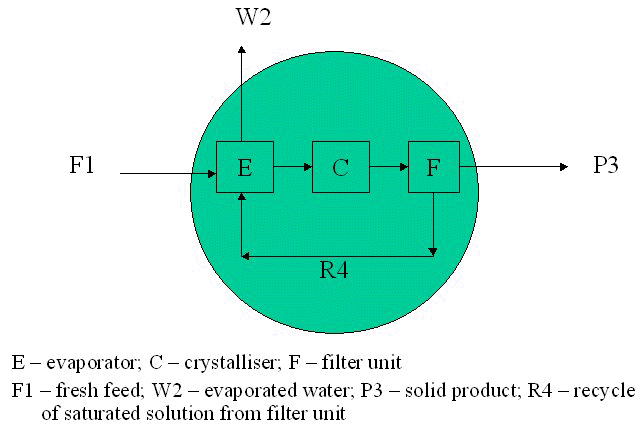Mass Balance Procedures
• Process description
• Flow sheet
• Label
• Assign algebraic symbols to unknowns (compositions, concentrations, quantities)
• Select basis
• Write mass balance equations (overall, total, component, unit) Solve equations for unknowns
Stoichiometry: Refers to quantities of reactants and products in a balanced chemical reaction.
aA + bB = cC + dD
a moles of A react with b moles of B to give c moles of C and d moles of D.
a,b,c,d are stoichiometric quantities.
Limiting Reactant / Excess Reactant: In practice a reactant may be used in excess of the stoichiometric quantity for various reasons. In this case the other reactant is limiting. It will limit the yield of product(s).
A reactant is in excess if it is present in a quantity greater than its stoichiometric proportion.
% excess = [(moles supplied – stoichiometric moles)/stoichiometric moles] x 100.
Conversion: Fractional conversion = amount reactant consumed/amount reactant supplied.
% conversion = fractional conversion x 100.
Note: conversion may apply to single pass reactor conversion or overall process conversion.
Yield = (moles product/moles limiting reactant supplied) x s.f. x 100.
Where s.f. is the stoichiometric factor = stoichiometric moles reactant required per mole of product.
Selectivity = (moles product/moles reactant converted) x s.f. x100.
OR
Selectivity = moles desired product/moles byproduct.
Extent of reaction = (moles of component leaving reactor – moles of component entering reactor)/stoichiometric coefficient of component.
Note: the stoichiometric coefficient of a component in a chemical reaction is the no. of moles in the balanced chemical equation ( -ve for reactants and +ve for products).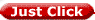to get all the information as a eBook